• Campus Map
• Directory
• Search

# Chem 10 - Common Lab Measurements

Here are the results for measuring Box 1 in the Common Lab Measurements lab in Chem 10. Please remember that for any measurement, there may be a small disagreement in the last digit of the measurement, but there should not be any disagreement about the number of decimal places or the overall value.

The table shows the values that should appear in the "Measure Box 1 here" table. Each measurement is explained in detail below the table.

dm ruler cm ruler mm ruler
width of box 1 1.6 dm 16.2 cm 161.5 mm
height of box 1 0.6 dm 6.5 cm 64.5 mm

## Measuring Box 1 using the dm ruler

In the picture below, you can see that the measurement of the width of box 1 falls between the "1" and "2" numbers on the decimeter ruler. Since we have already decided that we must measure the decimeter ruler to the tenths place, we know that the measurement will be between 1 and 2, so it will be 'one point something'. Now we just have to determine what 'something' is! Here we are using the fact that we also decided that we can break the smallest marked division into 10 imaginary divisions, making each imaginary division 0.1 dm. The red dashed "box width" line appears between the 1 and the 2, a bit to the right of half way between the numbers. Because "1.5" would be half way through, a good estimate of the width measurement would be "1.6 dm". A measurement of "1.7 dm" would also be reasonable. It is not OK to have a measurement of "1.70 dm" because that has too many digits after the decimal - it is not possible to read this ruler to the hundredths of dm.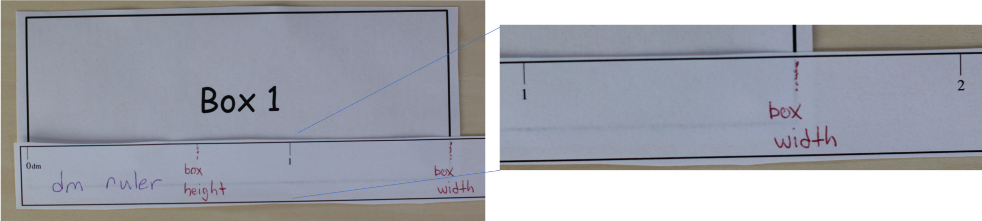In the picture below, you can see that the measurement of the height of box 1 falls between the "0" and "1" numbers on the decimeter ruler. Again, we are measuring to the tenths, since all measurements from the same measuring device have the same precision. Again, the dashed red line labeled "box height" appears just to the right of half way between 0 and 1, and a reasonable measurement for the height is "0.6 dm" or "0.7 dm".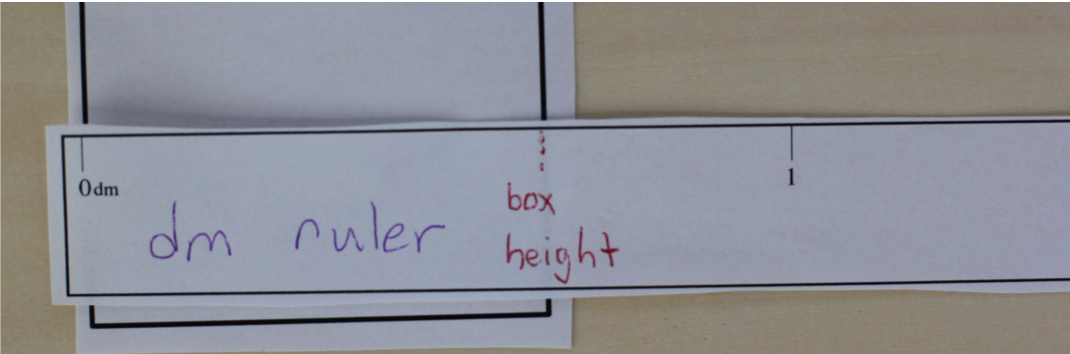## Measuring Box 1 using the cm ruler

In the picture below, you can see that the measurement of the width of box 1 falls between the "16" and "17" numbers on the centimeter ruler. Since we have already decided that we must measure the centimeter ruler to the tenths place, we know that the measurement will be between 16 and 17, so it will be '16 point something'. Now we just have to determine what 'something' is! Here we are using the fact that we also decided that we can break the smallest marked division into 10 imaginary divisions, making each imaginary division 0.1 cm. The red dashed "box width" line appears between the 16 and the 17, a bit to the right of the number 16, but not quite half way between 16 and 17. Because "16.5" would be half way through, a good estimate of the width measurement would be "16.2 cm". A measurement of "16.3 cm" would also be reasonable. It is not OK to have a measurement of "16.20 cm" because that has too many digits after the decimal - it is not possible to read this ruler to the hundredths of cm.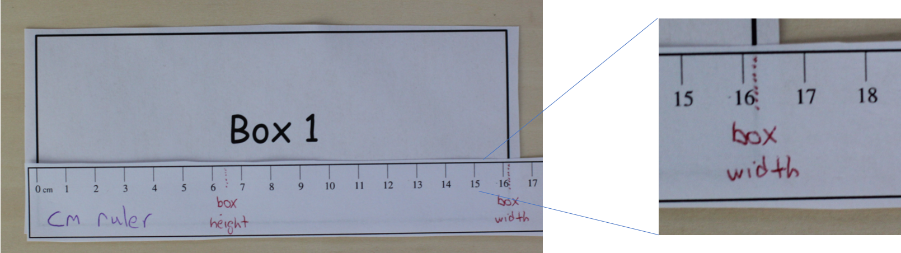In the picture below, you can see that the measurement of the height of box 1 falls between the "6" and "7" numbers on the centimeter ruler. Again, we are measuring to the tenths, since all measurements from the same measuring device have the same precision. The dashed red line labeled "box height" appears about half way between 6 and 7, and a reasonable measurement for the height is "6.5 cm".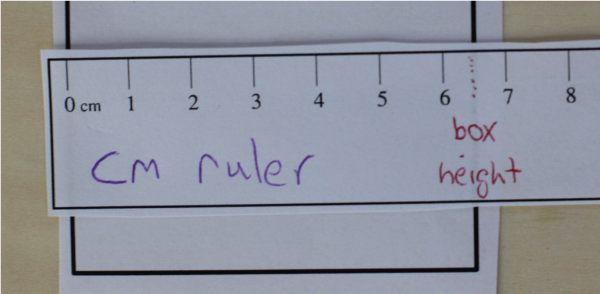## Measuring Box 1 using the mm ruler

In the picture below, you can see that the measurement of the width of box 1 falls between the "160" and "170" numbers on the millimeter ruler. However, we always want to look at where our measurement appears between the marks on a device, not on the numbers on a device. So we count over, and notice that the red dash is between the marks corresponding to "161" and "162". Since we have already decided that we must measure the millimeter ruler to the tenths place, we know that the measurement will be between 161 and 162, so it will be '161 point something'. Now we just have to determine what 'something' is!

The measurement gets slightly trickier here.

If you decided that you can only break the smallest marked divisions into 2 imaginary divisions, then your only choices for "point something" are "point zero" and "point five" (161.0 mm or 161.5 mm). You would specify 161.0 mm if the measurement appeared right on the 161 mm line, and 161.5 mm if it appeared between the two lines. In this case a measurement of "161.5 mm" would be most appropriate.

If you decided that you can only break the smallest marked divisions into 10 imaginary divisions, then you have to try and estimate where the mark appears. It is difficult to do in this case, but it still appears to be near the middle between "161" and "162", so a measurement of "161.5 mm" would be appropriate. However, since it was difficult to tell, another very reasonable scientist might say "161.4 mm", "161.6 mm", or even "161.3 mm" or "161.7 mm". All of these would be reasonable measurements of this box.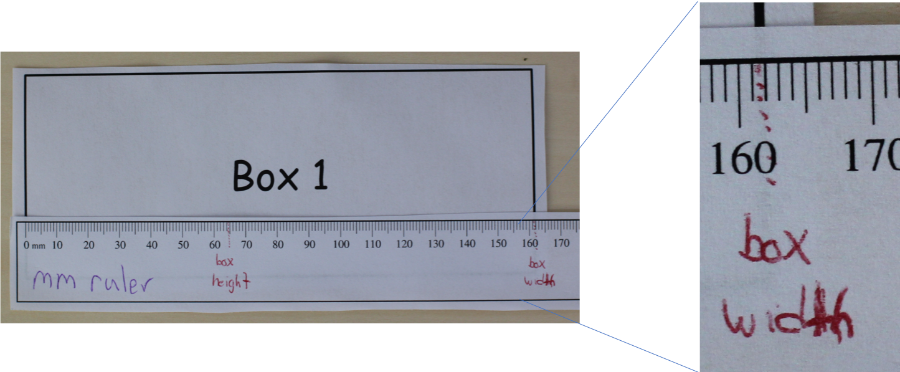In the picture below, you can see that the measurement of the height of box 1 falls between the "60" and "70" numbers on the millimeter ruler, but between the marks "64" and "65". Again, we are measuring to the tenths, since all measurements from the same measuring device have the same precision. The dashed red line labeled "box height" appears about half way between 64 and 65, and a reasonable measurement for the height is "64.5 mm".